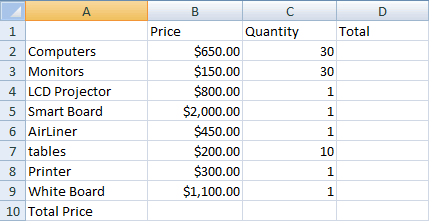﻿ Building a New Computer Lab

Building a New Computer Lab

 SOL CT 6-8.4 - 6-8.6, CT 6-8.8 SWBAT create a spreadsheet that explains the costs of creating a new computer lab at a school. Before What is a spreadsheet? Who uses spreadsheets? What do functions always start with? During Follow these directions to create a spreadsheet that explains the costs of creating a new computer lab at a school. Step 1 - Create the following spreadsheet:Step 2 - In Column D find the total cost for each item. This is the formula for multiplication: =(b2*c2). Step 3 - In cell D10 find the total cost for the lab. =sum(d2:d9) Step 4 - Create the following chart: You do not need to include column B or C, so hide them by right-clicking the columns letter and selecting Hide You do not need to include row 10After 1. What problems did you encounter? 2. As a total which item cost the most in the lab? 3. Create a Flow Map that explains how the total cost of the lab was calculated.